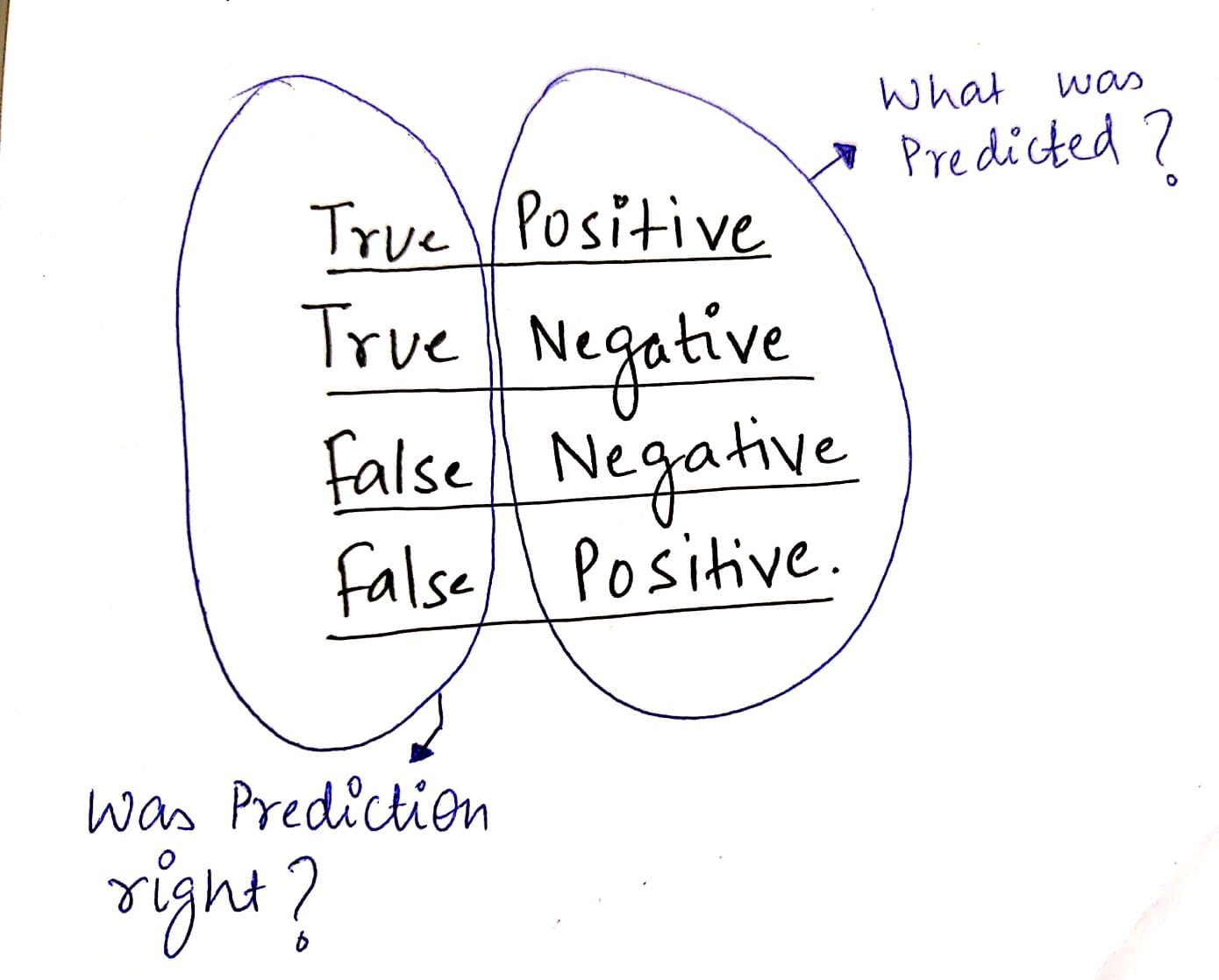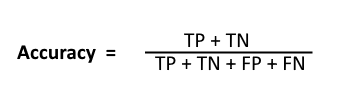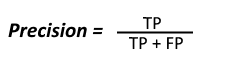1590173760

# Exploring confusion matrix evolution on tensorboard

Tensorboard is the best tool for visualizing many metrics while training and validating a neural network. In most of the case, we need to look for more details like how a model is performing on validation data. Sometimes training and validation loss and accuracy are not enough, we need to figure out the performance of validation data. One of the ways is to visualize using a confusion matrix.

#tensorflow #confusion-matrix #machine-learning

## Buddha Community1590173760

## Exploring confusion matrix evolution on tensorboard

Tensorboard is the best tool for visualizing many metrics while training and validating a neural network. In most of the case, we need to look for more details like how a model is performing on validation data. Sometimes training and validation loss and accuracy are not enough, we need to figure out the performance of validation data. One of the ways is to visualize using a confusion matrix.

#tensorflow #confusion-matrix #machine-learning1612593908

## Alpha Evolution Vital Keto Advanced Formula

Alpha Evolution Vital Keto is a natural and healthy fitness supplement for the body. This is the kind of supplement that people can use to make sure that they get the perfect fit. This supplement makes use of ketosis and the metabolism for getting the fat to be burnt away.

#alpha evolution keto #alpha evolution vital keto #alpha evolution vital keto reviews #alpha evolution keto canada #alpha evolution vital keto canada #alpha evolution reviews1597940460

## Confusion Matrix is no more confusing.

Before we switch into the topic, lets understand why we need to consider Confusion matrix and metrics ?

Metrics plays a major role in evaluating the performance of the model.

Metrics from Confusion Matrix.

• Confusion Matrix (Precision, Recall, F score, Accuracy)

Confusion Matrix is no more Confusing.

Consider a dataset has two classes say Class A and B. There may be two cases where your dataset is **Balanced **and Imbalanced. Balanced dataset means that, records for class A and B are balanced. Say Class A has 50% of data and class B has 50% of data or 55–45% of data. Imbalanced dataset has records of 90–10% of Class A and B or 80–20 and 70–30% of data.

Metrics to consider will be different for both Balanced and Imbalanced dataset.

Confusion Matrix comes with rows and columns of Actual and Predicted. The terminologies used are True Positive, True Negative, False positive, False Negative.

Lets split the words as True and positive separately.

Positive : Class A ; Negative : Not a Class A(Class B)

True : Predicted is right ; False : Predicted is wrongTrue Positive : _Positive _: Model predicted as Class A, **_True _**what model predicted is correct. Concludes as : Actual is Class A, Model Predicted as Class A.

True Negative : _Negative _: Model predicted as Class B, **_True _**what model predicted is correct.

#sklearn-metrics #confusion-matrix #metrics #precision-recall #imbalanced-data #data analytic1592936640

## Confusion Matrix is Not so Confusing

Confusion Matrix is a matrix that illustrates the performance of a classification model when exposed to unseen data. This matrix helps us to identify how the model is performing on test set. From this matrix, many other scores are calculated such as Accuracy, Recall, Precision, F1-score, etc. It is important one should know where to use which type of score as it depends on the application.

There are two classes: Class 1 and Class 2

Class 1:Positive

Class 2: Negative

Positive: Observation is True (eg. Picture is a dog)

Negative: Observation is False (eg. Picture is not a dog)

T.P.(True Positive): Truth and Prediction both are Positive

T.N.(True Negative): Truth and Prediction both are Negative

F.P.(False Positive): Truth is Negative but Prediction is Positive

F.N.(False Negative): Truth is Positive but Prediction is Negative

## Accuracy:

Accuracy is the ratio of sum of True Positive(T.P.) and True Negative(T.N.) to the sum of the matrix elements.## Precision:

Precision is defined as the ratio of True Positive(T.P) to the sum of True Positive(T.P) and False Positive(F.P)## Recall:

Recall is defined as the ratio of True Positive(T.P) to the sum of True Positive(T.P) and False Negative(F.N)_High recall, low precision: _This means that most of the positive examples are correctly recognized (low FN) but there are a lot of false positives.

_Low recall, high precision: _This shows that we miss a lot of positive examples (high FN) but those we predict as positive are indeed positive (low FP)

## F1-score:

Since we have two measures (Precision and Recall) it helps to have a measurement that represents both of them. We calculate an F-measure which uses Harmonic Mean in place of Arithmetic Mean as it punishes the extreme values more

#artificial-intelligence #python #confusion-matrix #machine-learning #data-science1582888330

## Learning to Design a School Performance Matrix | Simpliv

Description
The module “Designing School Performance Matrix” figures towards identification of important aspects towards Quality Initiatives in the schools on ground of Quality Requisites as a priority. The course modulates the connect towards important dimensions to the schools with contributions and recognition required to each in particular. The aspects relate parenting, classroom management, beneficiary satisfaction and the contribution to teaching learning practices in totality.

Who is the target audience?